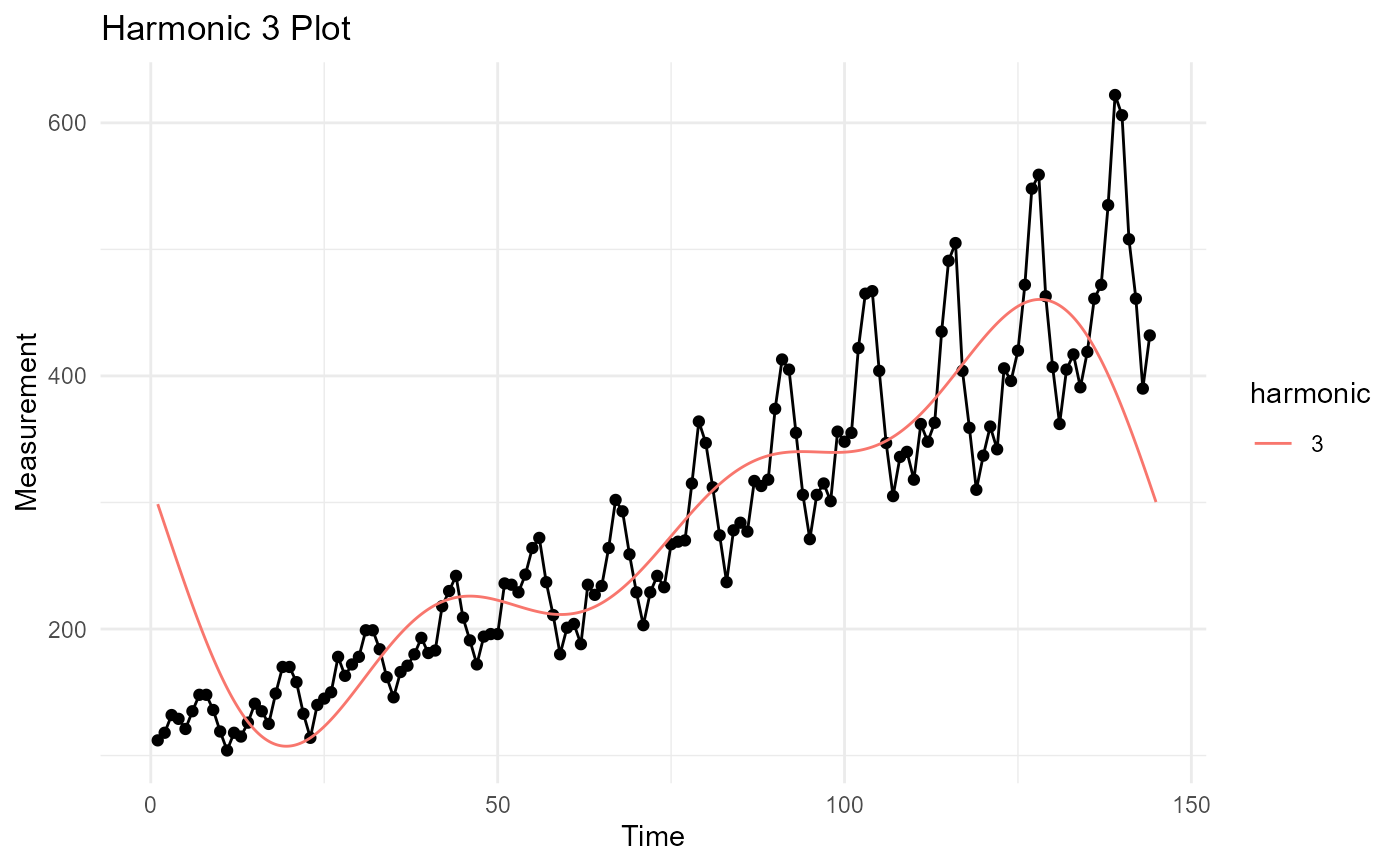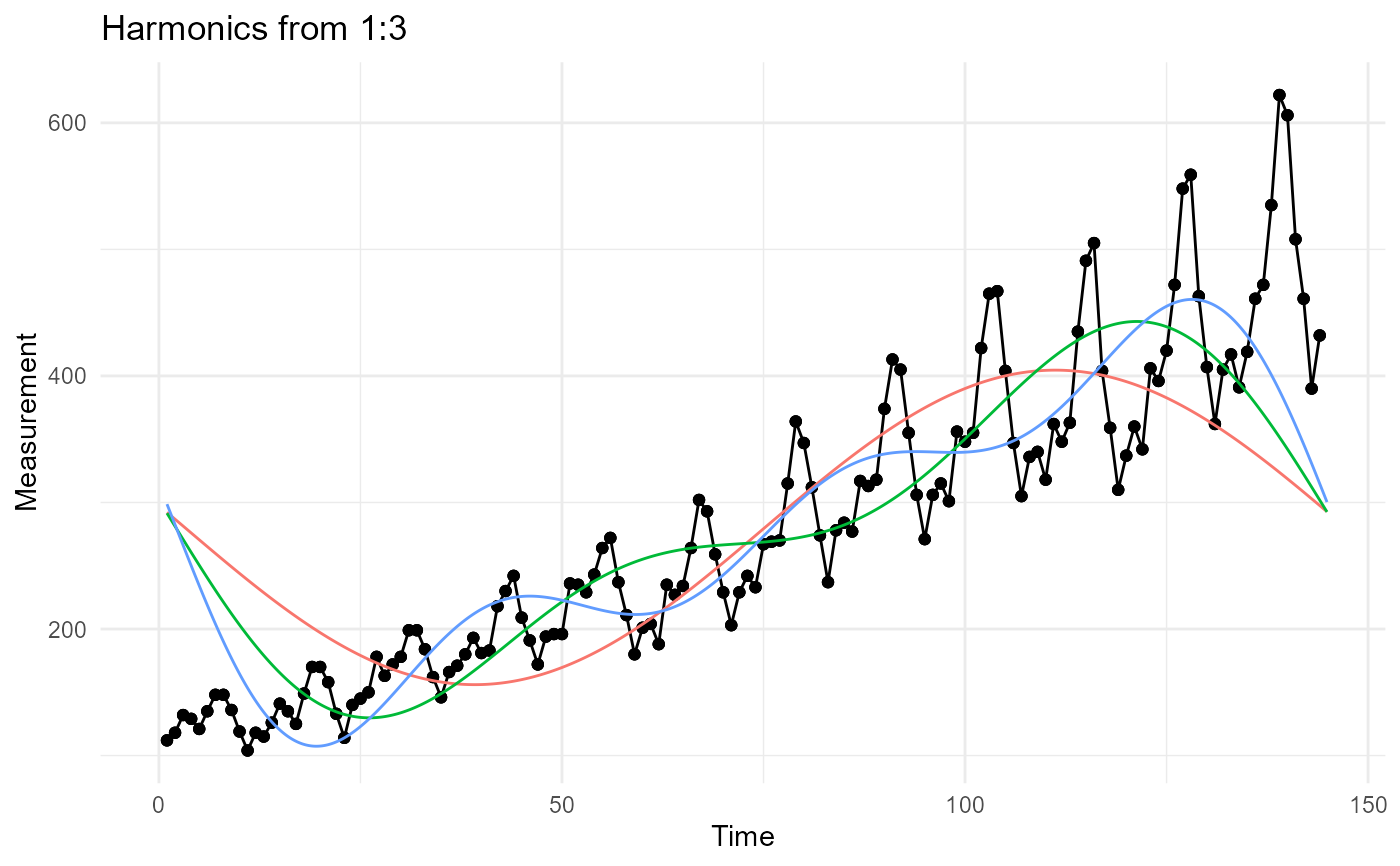Perform an fft using stats::fft() and return a tidier style output list with plots.

## Usage

tidy_fft(
.data,
.date_col,
.value_col,
.frequency = 12L,
.harmonics = 1L,
.upsampling = 10L
)

## Arguments

.data

The data.frame/tibble you will pass for analysis.

.date_col

The column that holds the date.

.value_col

The column that holds the data to be analyzed.

.frequency

The frequency of the data, 12 = monthly for example.

.harmonics

How many harmonic waves do you want to produce.

.upsampling

The up sampling of the time series.

## Value

A list object returned invisibly.

## Details

This function will perform a few different things, but primarily it will compute the Fast Discrete Fourier Transform (FFT) using stats::fft(). The formula is given as: $$y[h] = sum_{k=1}^n z[k]*exp(-2*pi*1i*(k-1)*(h-1)/n)$$

There are many items returned inside of a list invisibly. There are four primary categories of data returned in the list. Below are the primary categories and the items inside of them.

data:

1. data

2. error_data

3. input_vector

4. maximum_harmonic_tbl

5. differenced_value_tbl

6. dff_tbl

7. ts_obj

plots:

1. harmonic_plot

2. diff_plot

3. max_har_plot

4. harmonic_plotly

5. max_har_plotly

parameters:

1. harmonics

2. upsampling

3. start_date

4. end_date

5. freq

model:

1. m

2. harmonic_obj

3. harmonic_model

4. model_summary

Other Data Generator: ts_brownian_motion_augment(), ts_brownian_motion(), ts_geometric_brownian_motion_augment(), ts_geometric_brownian_motion(), ts_random_walk()

## Author

Steven P. Sanderson II, MPH

## Examples

suppressPackageStartupMessages(library(dplyr))

data_tbl <- AirPassengers %>%
ts_to_tbl() %>%
select(-index)

a <- tidy_fft(
.data = data_tbl,
.value_col = value,
.date_col = date_col,
.harmonics = 3,
.frequency = 12
)
#> Registered S3 methods overwritten by 'TSA':
#>   method       from
#>   fitted.Arima forecast
#>   plot.Arima   forecast

a$plots$max_har_plot
#> Warning: Removed 1296 rows containing missing values (geom_line()).
#> Warning: Removed 1296 rows containing missing values (geom_point()).a$plots$harmonic_plot
#> Warning: Removed 3888 rows containing missing values (geom_line()).
#> Warning: Removed 3888 rows containing missing values (geom_point()).